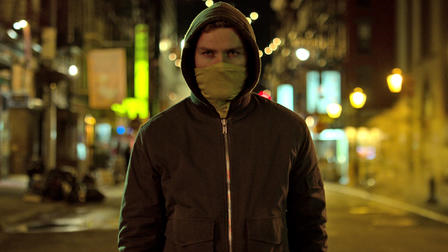October 9 2018

# Briefs And Phrases From Iron Fist- Season 2, Episode 1first time= FIRMT
last time= LAFMT
Cronuts= CROE/N*UTS
tiger= TAO*IRG
garlic= GLIK
shrimp= SHRIFRP
moo shu= MAOU/SHAO*U
midnight= MAOID
permanent= PERM
citizenship= SOIP
anime= AN/MA*I
culture= KLUR
kind of= KAOIF
sponge= SPUNG
Bob= BO*B
security guards= SGAURDZ
zone= SO*EN
nightclub= NAOIT/KL*UB
they happen= THEP
what this= WHATS
apologize= JAOIZ
center= STR-
besides= BESZ
last night= LAFNT
I got= *IGT
sideways= SDWAIS
misty= MIFT/YI
version= VERGS
takeout= TAIK/O*UT
Hester= HES/T*ER
good afternoon= GAFRN
shipment= SHIMT
passage= PAFJ
which has= KH-BS
what I have= WHAIF
customs= KUFMS
who go= WHOG
ravine= RA/VAO*EN
corpses= KORPSZ
measure= MUR
to tell= TOLT
cautionary= KAURGS
no more= NOERM
lady= LAED
karaoke= KAER/OEK/YI
solid= SLOD
adjustment= JAUFMT
cardinal= KARD/NA*L
milk= MIBLG
container= TAIRN
disgusting= SGUFGT
challenges= KHALS
practice= PRA
when the= WH-T
future= FAOURT
girlfriend= GOIF
armored= AORMD
golden= GOENLD
hatchet= HAFPT
territory= TERT
truces= TRAOUSZ
recruited= KRAOUTD
news= NUS
salary= SAL
partially= PARBL
grateful= GRAEF
Lana= LA/NA*
donation= DMAIGS
charity= KHAIRT
winter= WIRNT
treasure= TRERB
what do you think= WHAOUNG
about that= BHA
perfect= P-FRT
scarf= SKA*FR
have you got= V*UGT
Ethan= AOE/THA*N
we have= WEF
do you know= DAOUN
uptown= AUPT
Hudson= HUD/SO*N
New Yorker= NRORK
New York= NORK
Wisconsin= WIS/WIS
mediocre= MAOED/O*ERK
photograph= FOF
picture= PIR
camera= KMRA
vision= VIGS
magical= MIJ/KA*L
as much= S-FP
Mary= MA*ER
primer= PRAOIRM
pleasure= PLERB
Andrew= AN/DRAO*U
Albert= AL/B*ERT
starve= STAFRB
gallant= GLANT
hello= HO*EL
furniture= FURNT
cousin= KUFN
restaurant= STRAUNT
Henry= H*EN/RAO*E
bread= BRAED
out of= OUF
sufficient= SUF
crucial= KRAOURBL
barefoot= BAIR/FAO*T
as many= SMAEN
Broadway= BROI
hobbled= HOBLD
did you get= SDUGT
what is= WHAS
townhouse= TOUN/HO*US
technically= TLEBLG
official= FIRBL
to see= TOZ
is that= STHA
what this= WHATS
outside= OUDZ
divestment= DWEFMT
contract= KR-T
lump sum= LUMS
what you want= WHAUPT
expired= XAOIRD
patents= PA*ENTS
specific= SPEFK
ploy= PLO*I
benefit= BEF
punish= P-RB
about you= BU
nonsense= NAUNS
qualify= Q-F
to say= TO*S
all of this= AUFLTS
counter= KROURNT
reject= JEKT
proposal= PROEPL
we need= WEFRN
Harold= HA*RLD
all of it= A*UFLT
vetted= VETD
value= VAL
judgment= JUMT
mistake= MAEK
proud= PRO*UD
from you= FRU
some time= STAO*IM
lovely= LOFL
take care= TAERK
crazy= KRAEZ
beautiful= BAOUF
forwarding= FAORGD
crest= KREFT
donated= DMAITD
heirloom= HA*IR/LAO*M
I guess= IGS
when I first= WHIFRT
memory= MEM
must have been= MUFB
give me= GIFM
I mean= AO*IM
he said= EDZ
dinner= DIRN
opportunity= TAOUNT
to this= TOTS
how long= HOUNG
serenity= SER/R*ENT
months= MOS
intern= SBERN
negate= NA*EGT
pastries= PAIFT/TRAO*ES
physical= FIS
city= STI
fetid= FET/*ID
poison= POIFN
iniquity= IN/NIK/TAO*E
enjoy= GOI
wavering= WAFRG
dibs= DIBS
crab= KRAB
protein= PRAOEN
hairy= HAIR/YO
blood= BLAOD
lamb= LAM
insect= SNEKT
creativity= KRAOEFT
movie= MO*EF
admit= DMIT
you like= UBLG
why would= Y*LD
muscle= MUFL
Colleen= KO*L/LAO*EN
and I think= SKPING
power= POUR
vacuum= VAK/AO*UM
collide= KLAOID
Chinatown= KHAOIN/TO*UN
between the= TWAOENT
silver= SIFRL
lotus= LOE/T*US
this happen= TH-P
to give= TOFG
we got= W*EGT
meter= MAOERT
chef= SHEF
pork= PORK
bao= BAI/O*E
so much= SOFP
Tuesday= TAOUS
have you been= VUB
reasons= R-NS
I need= IFRN
simple= SIM
we’ve= WAO*EF
through this= THRUTS
ally= AL/LAO*I
where else= WRELS
company= K-P
mission= MIGS
destroy= STROI
there was= THR-FS
what I= WHAI
listening= L-NG
mastery= MAFRT/YI
do this= DO*TS
knowledge= NOJ
and you know= SKPUN
when you had= WHUD
did you= SDU
cowardice= KOURD/*IS
talk about= TAUB
if you could= FUKD
differently= DIFRNL
dragon= DRAG/O*N
I didn’t like= YIBLG
debatable= DB-T/-BL
what happened= WHAPD
duty= DAOUT
and you said= SKPUDZ
wasn’t= WAENT
I went= IFRG
mercy= MERZ
tonight= TONT
history= HIFT
manipulating= MIPG
ashes= ARBS

Tags: , , , ,
Copyright 2018. All rights reserved.

Posted October 9, 2018 by Elsie Villega in category "Briefs", "Steno Fun", "TV show